Practice the questions of McGraw Hill Math Grade 4 Answer Key PDF Chapter 8 Test to secure good marks &amp; knowledge in the exams.

Question 1.The Denominators of the given fractions are same so add the numerators.
3/5 + 1/5
= 3 + 1/5
= 4/5.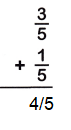Question 2.The Denominators of the given fractions are same so add the numerators.
1/8 + 5/8
= 6/8
= 3/4.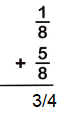Question 3.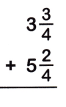3 x (3/4) = 15/4
5 x (2/4) = 22/4 = 11/2
Here the denominators are not equal. so, 15/4 + 11/2
= (15 x 2) + (11 x 4) ÷ 4 x 2
= 30+44/8
= 74/8
The 74/8 can be written has 9 x 1/4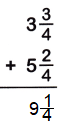Question 4.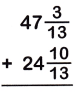47 x (3/13) = 614/13
24 x (10/13) = 322/13
The Denominators of the given fractions are same so add the numerators.
614/13 + 322/13 = 72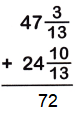Question 5.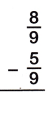The Denominators of the given fractions are same so subtract the numerators.
8/9 – 5/9
= 8-5/9
= 3/9
= 1/3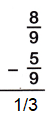Question 6.The Denominators of the given fractions are same so subtract the numerators.
9/10 – 4/10
= 9-4/10
= 5/10
= 1/2Question 7.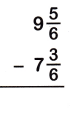9 x (5/6) = 59/6
7 x (3/6) = 45/6
The Denominators of the given fractions are same so subtract the numerators.
59/6 – 45/6
= 59-45/6
= 14/6
= 7/3
7/3 can be written as 2 x 1/3.Question 8.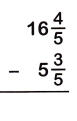5 x (3/5) = 54/5
16 x (4/5) =28/5
The Denominators of the given fractions are same so subtract the numerators.
54/5 – 28/5
= 54-28/5
= 26/5
26/5 can be written has 5 x 1/5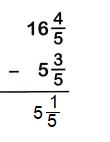Question 9.The Denominators of the given fractions are same so add the numerators.
8/16 + 5/16
= 8+5/16
= 13/16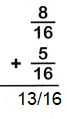Question 10.The Denominators of the given fractions are same so subtract the numerators.
12/14 – 8/14
= 12-8/14
= 4/14
= 2/7Question 11.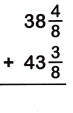38 x (4/8) = 308/8
43 x (3/8) = 347/8
The Denominators of the given fractions are same so add the numerators.
308/8 + 347/8
= 308+347/8
= 655/8
= 81 x 7/8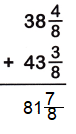Question 12.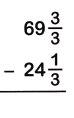69 x (3/3) = 210/3
24 x (1/3) = 73/3
The Denominators of the given fractions are same so subtract the numerators.
210/3 – 73/3
= 210-73/3
= 137/3
= 45 x 2/3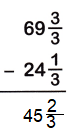Question 13.
A family bought apples at a farmer’s market. They bought a bag of red apples that weighed 7$$\frac{4}{8}$$ pounds and a bag of green apples that weighed 3$$\frac{3}{8}$$ pounds on Saturday. How many pounds of apples did they buy?
The family bought a bag of red apples = 7 x (4/8) pounds = 60/8
The family bought a bag of green apples = 3 x (3/8) pounds = 27/8
The Denominators of the given fractions are same so add the numerators.
60/8 + 27/8 = 60-27/8
= 87/8
= 10 x 7/8

Hence, the total number of apples that the family bought is 2 x (7/8) pounds.

Question 14.
Alex makes a salad for the family. He uses 4$$\frac{2}{9}$$ cups of lettuce, 1$$\frac{1}{9}$$ cups of tomatoes, and 2$$\frac{4}{9}$$ cups of cucumbers. How many more cups of lettuce does he use than cucumbers?
Given that,
The total number of lettuce cups = 4 x (2/9) cups = 38/9.
The total number of tomato cups = 1 x (1/9) cups = 10/9.
The total number of cucumber cups = 2 x (4/9) cups = 22/9.
Therefore the lettuce cups more than cucumber cups is 38/9 – 22/9
The Denominators of the given fractions are same so subtract the numerators.
= 38-22/9
= 16/9
= 1 x 7/9 cups.
Hence, the number of lettuce cups more than the cucumber cups is 1 x 7/9 cups.

Question 15.
Write $$\frac{4}{9}$$ as a sum of fractions.
Given that the fraction is 4/9.
The sum of the fraction of 4/9 is 1/9 + 1/9 + 1/9 + 1/9.

Question 16.
Write 1$$\frac{3}{5}$$ as a sum of fractions.
Given that the mixed fraction is 1 x (3/5)
1 x (3/5) = 8/5
The sum of the fractions of 8/5 is 1/5 + 1/5 + 1/5 + 1/5 + 1/5 + 1/5 + 1/5 +1/5.

Multiply. Write your answers in simplest form. Draw pictures if you need help.

Question 17.
4 × $$\frac{1}{3}$$ = ______________
Multiply 4 x 1/3 then you get 4/3

Question 18.
5 × $$\frac{3}{4}$$ = ______________
Multiply 5 x 3/4 then you get 23/4

Question 19.
3 × $$\frac{3}{5}$$ = ______________
Multiply 3 x 3/5 then you get 18/5

Question 20.
7 × $$\frac{4}{8}$$ = ______________
Multiply 7 x 4/8 then you get 60/8.

Question 21.
Jason is practicing for a footrace. He runs 7$$\frac{3}{8}$$ miles the first week and 9$$\frac{6}{8}$$ miles the second week. How many miles does Jason run in all?
Given that,
Jason runs in the first week = 7 x (3/8) = 59/8
Jason runs in the second week = 9 x (6/8) = 78/8
The Denominators of the given fractions are same so add the numerators.
59/8 + 78/8
= 59+78/8
= 137/8
= 17 x 1/8
Hence, Jason run in all is 17 x 1/8 miles.

Question 22.
76 vehicles are in a parking lot. $$\frac{1}{4}$$ of them are trucks. How many trucks are in the parking lot?
Given that,
The total number of vehicles in the parking is 76.
The number of trucks in the parking lot is $$\frac{1}{4}$$ = 1/4
Therefore 76 x 1/4 = 19.
The total number of trucks in the parking lot is 19.

Question 23.
A hardware store sold 7$$\frac{8}{10}$$ feet of chain in one week. The store sold 4$$\frac{3}{10}$$ feet of chain the next week. How many more feet of chain did the store sell in the first week?
Given that,
The hardware store sold a chain in one week = 7 x (8/10) = 78/10.
The hardware store sold a chain in next week = 4 x (3/10) = 43/10.
The Denominators of the given fractions are same so subtract the numerators.
78/10 – 43/10
= 78-43/10
= 35/10
= 7/2
= 3 x 1/2
Hence, the chain sold in one week is 2 x (1/2) feet’s longer then chain sold in next week.

Question 24.
Linda travels to visit friends. She drives for a total of 23 miles. Damon also visits friends, but his trip is $$\frac{2}{3}$$ as long as Linda’s. How many miles does Damon drive?
Damon also visits friends, but his trip is $$\frac{2}{3}$$ as long as Linda’s.
$$\frac{2}{3}$$ = 2/3